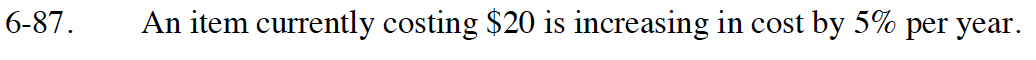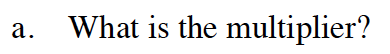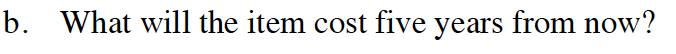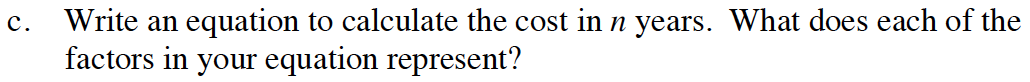Home > GB8I > Chapter cc46 > Lesson cc46.3.1 > Problem6-87

6-87.
1. An item currently costing $20 is increasing in cost by 5% per year. Homework Help ✎ 1. What is the multiplier? 2. What will the item cost five years from now? 3. Write an equation to calculate the cost in n years. What does each of the factors in your equation represent?The cost is increasing, so the multiplier will be greater than 1. 1.05The original cost would be multiplied by 1.05 for the first year, an additional 1.05 for the second year, an additional 1.05 every year after that.$25.52Use the starting value and multiplier to write an equation of the form t(n) = abn